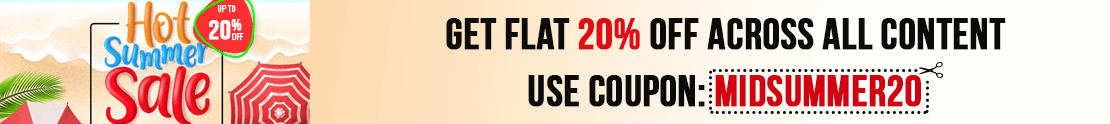# User ForumSubject :IMO    Class : Class 6

## Ans 1:

Class : Class 9
Solution=Let the quantity of water in bottleP = 3x and that of in bottle Q = 4x.According to question,â 20(3x â 40) = 4x Ã 13â 60x â 800 = 52x â 8x = 800 â x = 100So, quantity of water in bottle P = 3 Ã 100= 300 mLans.-c

## Ans 2:

Class : Class 6
C

Subject :IMO    Class : Class 8

Subject :IMO    Class : Class 5

## Ans 1:

Class : Class 5
B cause the rule is based on multiplication 0 input will be 0 as out put cause if you multiply 0 by 0 you get 0 and other answers don't have that as an option

Subject :IMO    Class : Class 5

Subject :IMO    Class : Class 5

## Ans 1:

Class : Class 8
b

Subject :IMO    Class : Class 6

## Ans 1:

Class : Class 6
it is b since 114-6=108

## Ans 2:

Class : Class 4
The answer is definitely 108 me calculator everyone prooves it kindly improve it

Subject :IMO    Class : Class 5

## Ans 1:

Class : Class 8
The answer is D . Because 1460-50 g =1410 g. 1410/30=47. so 47*40=18.80. 18.80 + 1.50. =20.30 ans

Subject :IMO    Class : Class 8

Subject :IMO    Class : Class 5